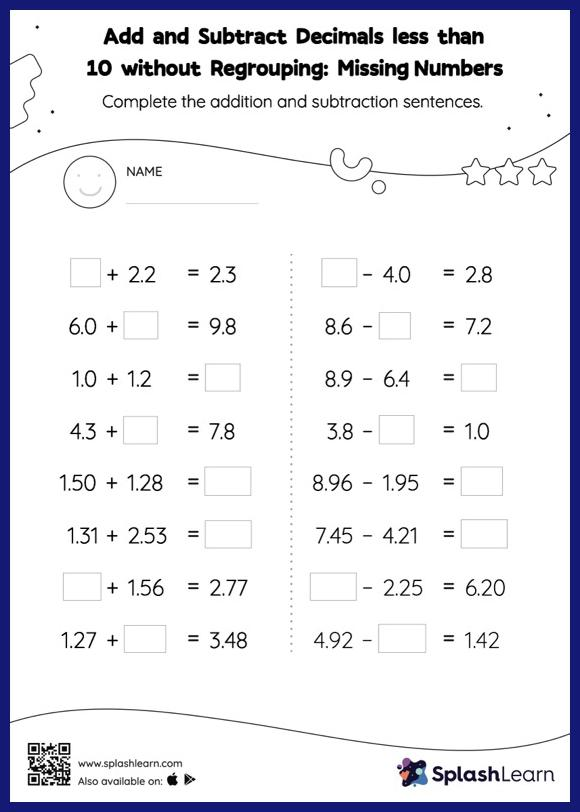# Add and Subtract Decimals less than 10 without Regrouping: Missing Numbers Worksheet

Home > Add and Subtract Decimals less than 10 without Regrouping: Missing NumbersStudents use the relationship between addition and subtraction to find the missing number while adding and subtracting decimals. They do not need to regroup the numbers in add and subtract decimals less than 10 without regrouping worksheet to get to the result. This worksheet is about practicing with the horizontal format in which numbers are written side by side. To develop flexibility with numbers and operations, students need to have enough practice in this format and not just rely on the vertical/column method.